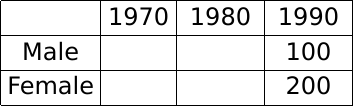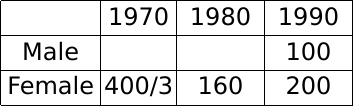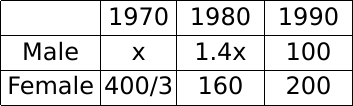# CAT Ratio and Proportion Questions PDF [Most Important with Solutions]

0
872

Ratio and Proportions for CAT is an important topic in the Quant section. Over the past few years, Ratio and Proportion have made a recurrent appearance in the CAT Quants Section. You can expect around 2-3 questions in the 22-question format of the CAT Quant sections. You can check out these Ratio and Proportion CAT Previous year questions.  In this article, we will look into some important Ratio & Proportions Questions for CAT. These are a good source for practice; If you want to practice these questions, you can download this CAT Ratios and Proportions Questions PDF, which is completely Free.

• CAT Ratios & Proportions – Tip 1: CAT Ratio and Proportion questions appear in the CAT and other MBA entrance exams every year. If you’re starting the prep, firstly understand the CAT Arithmetic Syllabus; It is one of the most important topics and hence should not be avoided. Based on our analysis of the Arithmetic CAT Previous Year Questions: 3-4 questions were asked on this topic.
• CAT Ratios & Proportions – Tip 2: In the CAT exam, CAT Ratio questions are Not very tough. A strong foundation in this topic will aid a student in answering these questions. Practice these CAT Ratio and Proportion problems with solutions PDF. Getting yourself acquainted with the basics of these concepts will help you solve the problems. Learn all the major formulae from these concepts. You can learn all the Important CAT Ratio & Proportion Formulas here.

Question 1: If a certain weight of an alloy of silver and copper is mixed with 3 kg of pure silver, the resulting alloy will have 90% silver by weight. If the same weight of the initial alloy is mixed with 2 kg of another alloy which has 90% silver by weight, the resulting alloy will have 84% silver by weight. Then, the weight of the initial alloy, in kg, is

a) 3.5

b) 2.5

c) 3

d) 4

Solution:

Let the alloy contain x Kg silver and y kg copper
Now when mixed with 3Kg Pure silver
we get $\frac{\left(x+3\right)}{x+y+3}=\frac{9}{10}$
we get 10x+30 =9x+9y+27
9y-x=3    (1)
Now as per condition 2
silver in 2nd alloy = 2(0.9) =1.8
so we get$\frac{\left(x+1.8\right)}{x+y+2}=\frac{21}{25}$
we get 21y-4x =3   (2)
solving (1) and (2) we get y= 0.6 and x =2.4
so x+y = 3

Question 2: A tea shop offers tea in cups of three different sizes. The product of the prices, in INR, of three different sizes is equal to 800. The prices of the smallest size and the medium size are in the ratio 2 : 5. If the shop owner decides to increase the prices of the smallest and the medium ones by INR 6 keeping the price of the largest size unchanged, the product then changes to 3200. The sum of the original prices of three different sizes, in INR, is

Solution:

Let price of smallest cup be 2x and medium be 5x and large be y
Now by condition  1
we get $2x\ \times\ \ 5x\ \times\ y\ =800$
we get $x^2y\ =80$    (1)
Now as per second condition ;
$\left(2x+6\right)\times\ \left(5x+6\right)\ y\ =3200$        (2)
Now dividing (2) and (1)
we get $\frac{\left(\left(2x+6\right)\times\ \left(5x+6\right)\right)}{x^2}=40$
we get $10x^2+42x+36\ =\ 40x^2$
we get $\ 30x^2-42x-36=0$
$5x^2-7x-6=0$
we get x=2
So 2x=4 and 5x=10
Now substituting in (1) we get y =20
Now therefore sum = 4+10+20 =34
Question 3: One part of a hostel’s monthly expenses is fixed, and the other part is proportional to the number of its boarders. The hostel collects ₹ 1600 per month from each boarder. When the number of boarders is 50, the profit of the hostel is ₹ 200 per boarder, and when the number of boarders is 75, the profit of the hostel is ₹ 250 per boarder. When the number of boarders is 80, the total profit of the hostel, in INR, will be

a) 20200

b) 20500

c) 20800

d) 20000

Solution:

Profit per boarder = Total profit / Number of boarders.

Let the number of boarders be n.

Profit/boarder = 1600 – (Total cost/n)

Let the total cost be a + bn, where a = fixed, and b is the variable additional cost per boarder.

Profit/boarder = 1600 – (a + bn)/n

Profit/boarder = 1600 – a/n – b

1600 – a/50 – b = 200

1600 – a/75 – b = 250

Solving, we get a = 7500, and b = 1250

Hence, total profit with 80 people = 80 ( 1600 – 7500/80 – 1250) = 80 (350 – 7500/80) = 28000 – 7500 = Rs. 20500

Question 4: The arithmetic mean of scores of 25 students in an examination is 50. Five of these students top the examination with the same score. If the scores of the other students are distinct integers with the lowest being 30, then the maximum possible score of the toppers is

Solution:

Let sum of marks of students be x
Now therefore x = 25*50 =1250
Now to maximize the marks of the toppers
We will minimize the marks of 20 students
so their scores will be (30,31,32…..49 )
let score of toppers be y
so we get 5y +$\frac{20}{2}\left(79\right)$=1250
we get 5y +790=1250
5y=460
y=92
So scores of toppers = 92

Question 5: The total of male and female populations in a city increased by 25% from 1970 to 1980. During the same period, the male population increased by 40% while the female population increased by 20%. From 1980 to 1990, the female population increased by 25%. In 1990, if the female population is twice the male population, then the percentage increase in the total of male and female populations in the city from 1970 to 1990 is

a) 68.25

b) 68.75

c) 68.50

d) 69.25

Solution:

Let us solve this question by assuming values(multiples of 100) and not variables(x).

Since we know that the female population was twice the male population in 1990, let us assume their respective values as 200 and 100.

Note that while assuming numbers, some of the population values might come out as a fraction(which is not possible, since the population needs to be a natural number). However, this would not affect our answer, since the calculations are in ratios and percentages and not real values of the population in any given year.Now, we know that the female population became 1.25 times itself in 1990 from what it was in 1980.

Hence, the female population in 1980 = 200/1.25 = 160

Also, the female population became 1.2 times itself in 1980 from what it was in 1970.

Hence, the female population in 1970 = 160/1.2 = 1600/12 = 400/3Let the male population in 1970 be x. Hence, the male population in 1980 is 1.4x.Now, the total population in 1980 = 1.25 times the total population in 1970.

Hence, 1.25 (x + 400/3) = 1.4x + 160

Hence, x = 400/9.

Population change = 300 – 400/9 – 400/3 = 300 – 1600/9 = 1100/9

percentage change = $\frac{\frac{1100}{9}}{\frac{1600}{9}}\times\ 100\ =\ \frac{1100}{16}\%=68.75\%$

Question 6: In a tournament, a team has played 40 matches so far and won 30% of them. If they win 60% of the remaining matches, their overall win percentage will be 50%. Suppose they win 90% of the remaining matches, then the total number of matches won by the team in the tournament will be

a) 80

b) 78

c) 84

d) 86

Solution:

Initially number of matches = 40
Now matches won = 12
Now let remaining matches be x
Now number of matches won = 0.6x
Now as per the condition :
$\frac{\left(12+0.6x\right)}{40+x}=\frac{1}{2}$
24 +1.2x=40+x
0.2x=16
x=80
Now when they won 90% of remaining = 80(0.9) =72
So total won = 84

Question 7: A person buys tea of three different qualities at ₹ 800, ₹ 500, and ₹ 300 per kg, respectively, and the amounts bought are in the proportion 2 : 3 : 5. She mixes all the tea and sells one-sixth of the mixture at ₹ 700 per kg. The price, in INR per kg, at which she should sell the remaining tea, to make an overall profit of 50%, is

a) 653

b) 688

c) 692

d) 675

Solution:

Considering the three kinds of tea are A, B, and C.

The price of kind A = Rs 800 per kg.

The price of kind B = Rs 500 per kg.

The price of kind C = Rs 300 per kg.

They were mixed in the ratio of 2 : 3: 5.

1/6 of the total mixture is sold for Rs 700 per kg.

Assuming the ratio of mixture to A = 12kg, B = 18kg, C =30 kg.

The total cost price is 800*12+500*18+300*30 = Rs 27600.

Selling 1/6 which is 10kg for Rs 700/kg the revenue earned is Rs 7000.

In order to have an overall profit of 50 percent on Rs 27600.

Thes selling price of the 60 kg is Rs 27600*1.5 = Rs 41400.

Hence he must sell the remaining 50 kg mixture for Rs 41400 – Rs 7000 = 34400.

Hence the price per kg is Rs 34400/50 = Rs 688

Question 8: In a football tournament, a player has played a certain number of matches and 10 more matches are to be played. If he scores a total of one goal over the next 10 matches, his overall average will be 0.15 goals per match. On the other hand, if he scores a total of two goals over the next 10 matches, his overall average will be 0.2 goals per match. The number of matches he has played is

Solution:

Let Total matches played be n and in initial n-10 matches his goals be x
so we get $\frac{\left(x+1\right)}{n}=0.15$
we get x+1 =0.15n                (1)
From condition (2) we get :
$\frac{\left(x+2\right)}{n}=0.2$
we get x+2 = 0.2n                  (2)
Subtracting (1) and (2)
we get 1 =0.05n
n =20
So initially he played n-10 =10 matches

Question 9: A box has 450 balls, each either white or black, there being as many metallic white balls as metallic black balls. If 40% of the white balls and 50% of the black balls are metallic, then the number of non-metallic balls in the box is

Solution:

Let the number of white balls be x and black balls be y
So we get x+y =450       (1)
Now metallic black balls = 0.5y
Metallic white balls = 0.4x
From condition 0.4x=0.5y
we get 4x-5y=0     (2)
Solving (1) and (2) we get
x=250 and y =200
Now number of Non Metallic balls = 0.6x+0.5y = 150+100 = 250

Question 10: From a container filled with milk, 9 litres of milk are drawn and replaced with water. Next, from the same container, 9 litres are drawn and again replaced with water. If the volumes of milk and water in the container are now in the ratio of 16 : 9, then the capacity of the container, in litres, is

Solution:

Let initial volume be V, final be F for milk.

The formula is given by : $F\ =\ V\cdot\left(1-\frac{K}{V}\right)^n$ n is the number of times the milk is drawn and replaced.

so we get $F=\ V\left(1-\frac{K}{V}\right)^{^2}$
here K =9
we get
$\frac{16}{25}V\ =\ V\ \left(1-\frac{9}{V}\right)^{^2}$
we get $1-\frac{9}{V}=\ \frac{4}{5}or\ -\frac{4}{5}$

If considering $1-\frac{9}{V}=-\frac{4}{5}$

V =5, but this is not possible because 9 liters is drawn every time.

Hence : $1-\frac{9}{V}=\frac{4}{5},\ V\ =\ 45\ liters$

Check out the CAT Formula Handbook which includes the most important formulas you must know for CAT.
• So, these are some of the most important CAT Ratio questions PDF. Download these Ratio & Proportions Questions for CAT PDF, with detailed Answers. Also, practice the ratio and proportion concepts well; it is not a tough topic.
• Practising and solving these questions and answers is one of the key steps in acing CAT Arithmetic questions. You can also check out CAT Ratio & Proportions Previous year Questions with detailed solutions here.
• Try these 3 Cracku Free CAT Mocks, which come with detailed solutions and with video explanations.